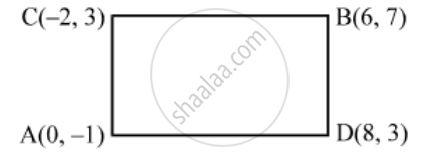# Show that Four Points (0, – 1), (6, 7), (–2, 3) and (8, 3) Are the Vertices of a Rectangle. Also, Find Its Area - Mathematics

Sum

Show that four points (0, – 1), (6, 7), (–2, 3) and (8, 3) are the vertices of a rectangle. Also, find its area

#### Solution

Let A (0, –1), B(6, 7), C(–2, 3) and D (8, 3) be the given points. Then,

AD=sqrt((8-0)^2+(3+1)^2)=\sqrt{64+16}=4\sqrt{5}

BC=sqrt((6+2)^2+(7+3)^2)=\sqrt{64+16}=4\sqrt{5}

AC=sqrt((-2-0)^2+(3+1)^2)=\sqrt{4+16}=2\sqrt{5}

and,\text{ }BD\text{}=sqrt((8-6)^2+(3-7)^2)=\sqrt{4+16}=2\sqrt{5}

∴ AD = BC and AC = BD.Now,\text{ }AB\text{ }=sqrt((6-0)^2+(7+1)^2)=\sqrt{36+64}=10

Clearly,  AB^2 = AD^2 + DB^2 and CD^2 = CB^2 + BD^2

= (4√5 × 2√5 ) sq. units = 40 sq. units

Concept: Distance Formula
Is there an error in this question or solution?